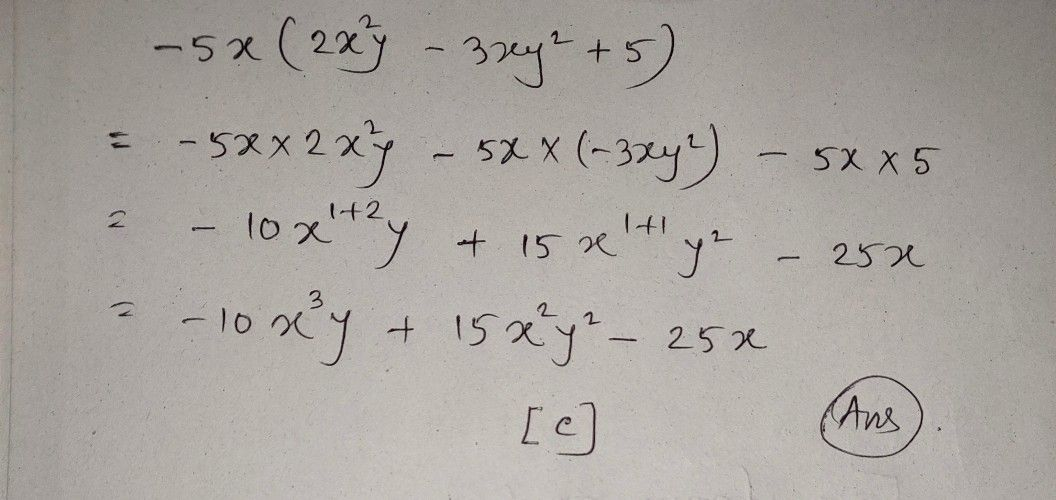Symbol
ProblemMultiply. - $5x\left(2x^{2}y-3y^{2}+5\right)$ $○$ $A-10x^{3}y-15x^{2}y^{2}-25x$ $○$ $B$ $-10x^{2}y+15xy^{2}-25x$ $\square$ $C-10x^{3}y+15x^{2}y^{2}-25x$ $\square$ O $-$ $10x^{2}y-15xy^{2}-25x$